# 图像阈值

• 固定阈值，自适应阈值，Otsu 二值化等
• 全局阈值和局部阈值

## 一、图像二值化

• 定义：图像的二值化，就是将图像上的像素点的灰度值设置为0或255，也就是将整个图像呈现出明显的只有黑和白的视觉效果。

• 灰度值0：黑，灰度值255：白

• 一幅图像包括目标物体、背景还有噪声，要想从多值的数字图像中直接提取出目标物体，常用的方法就是设定一个阈值T，用T将图像的数据分成两部分：大于T的像素群和小于T的像素群。这是研究灰度变换的最特殊的方法，称为图像的二值化（Binarization）。

## 二、固定阈值

cv2.threshold(img, threshold, maxval,type)

• 参数解释：
• img就是原图像，原图像应该是灰度图
• threshold是设定的阈值，就是用来对像素值进行分类的阈值
• maxval是当灰度值大于（或小于）阈值时将该灰度值赋成的值
• type规定的是当前二值化的方式

OpenCV提供了多种不同的阈值方法，这是有第四个参数来决定的。这些方法包括：

• cv2.THRESH_BINARY
• 二值化阈值，大于阈值的部分被置为255，小于部分被置为0
• cv2.THRESH_BINARY_INV
• 反向二值化阈值，大于阈值部分被置为0，小于部分被置为255
• cv2.THRESH_TRUNC
• 截断阈值化，大于阈值部分被置为threshold，小于部分保持原样
• cv2.THRESH_TOZERO
• 小于阈值部分被置为0，大于部分保持不变
• cv2.THRESH_TOZERO_INV
• 大于阈值部分被置为0，小于部分保持不变
import cv2
import numpy as np
from matplotlib import pyplot as plt

ret,thresh1=cv2.threshold(img,127,255,cv2.THRESH_BINARY)
ret,thresh2=cv2.threshold(img,127,255,cv2.THRESH_BINARY_INV)
ret,thresh3=cv2.threshold(img,127,255,cv2.THRESH_TRUNC)
ret,thresh4=cv2.threshold(img,127,255,cv2.THRESH_TOZERO)
ret,thresh5=cv2.threshold(img,127,255,cv2.THRESH_TOZERO_INV)

titles = ['Original Image','BINARY','BINARY_INV','TRUNC','TOZERO','TOZERO_INV']
images = [img, thresh1, thresh2, thresh3, thresh4, thresh5]

plt.figure(figsize = (20, 10))
for i in range(6):
plt.subplot(2,3,i+1)
plt.imshow(images[i],'gray')
plt.title(titles[i])
plt.xticks([]),plt.yticks([])

plt.show()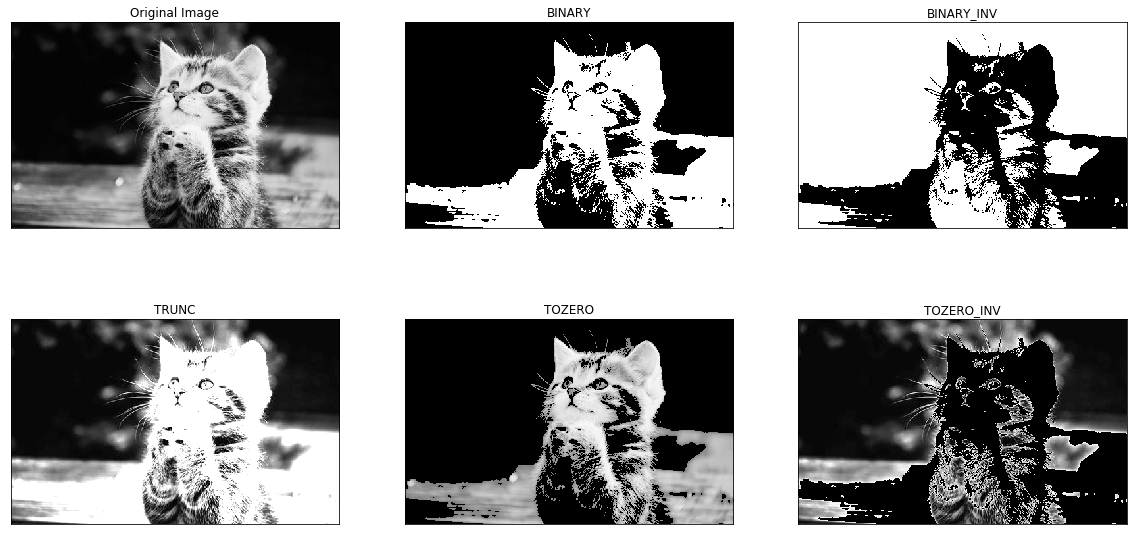## 三、自适应阈值

1. 在图像阈值化操作中，更关注的是从二值化图像中，分离目标区域和背景区域，但是仅仅通过设定固定阈值很难达到理想的分割效果。

在前面的部分我们使用是全局阈值，整幅图像采用同一个数作为阈值。当时这种方法并不适应与所有情况，尤其是当同一幅图像上的不同部分的具有不同亮度时。这种情况下我们需要采用自适应阈值。此时的阈值是根据图像上的每一个小区域计算与其对应的阈值。因此在同一幅图像上的不同区域采用的是不同的阈值，从而使我们能在亮度不同的情况下得到更好的结果。

2. 自适应阈值，则是根据像素的邻域块的像素值分布来确定该像素位置上的二值化阈值。这样做的好处

• 每个像素位置处的二值化阈值不是固定不变的，而是由其周围邻域像素的分布来决定的。

• 亮度较高的图像区域的二值化阈值通常会较高，而亮度低的图像区域的二值化阈值则会相适应的变小。

• 不同亮度、对比度、纹理的局部图像区域将会拥有相对应的局部二值化阈值。

• 适合处理光照不均的图像。

• thresholdType 指定阈值类型。可选择THRESH_BINARY或者THRESH_BINARY_INV两种。（即二进制阈值或反二进制阈值）

• Block Size 邻域大小（用来计算阈值的区域大小），一般选择为3、5、7…等。

• C 参数C表示与算法有关的参数，阈值就等于的平均值或者加权平均值减去这个常数，可以是负数。

4. 自适应阈值化计算过程

自适应阈值化计算大概过程是为每一个象素点单独计算的阈值，即每个像素点的阈值都是不同的，就是将该像素点周围B*B区域内的像素加权平均，然后减去一个常数C，从而得到该点的阈值。

 - ADAPTIVE_THRESH_MEAN_C，为局部邻域块的平均值，该算法是先求出块中的均值，再减去常数C。

5. 例子（参考博客）

• 如果使用平均值方法，平均值mean为190，差值delta（即常数C）为30。那么灰度小于160的像素为0，大于等于160的像素为255。

• 二值化：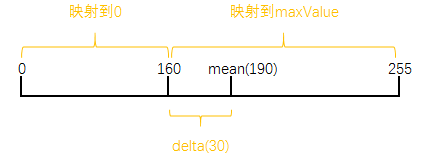• 反向二值化：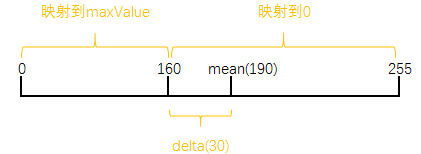import cv2
import numpy as np
from matplotlib import pyplot as plt

# 中值滤波  做不做滤波处理等对图像分割影响也比较大，感兴趣的可以自己测试一下。
gray = cv2.medianBlur(gray,5)

ret,th1 = cv2.threshold(gray,127,255,cv2.THRESH_BINARY)

titles = ['Original Image', 'Gray Image','Global Thresholding (v = 127)','Adaptive Mean Thresholding', 'Adaptive Gaussian Thresholding']
images = [img, gray, th1, th2, th3]

plt.figure(figsize = (20, 15))
for i in range(5):
plt.subplot(1,5,i+1)
plt.imshow(images[i],'gray')
plt.title(titles[i])
plt.xticks([]),plt.yticks([])
plt.show()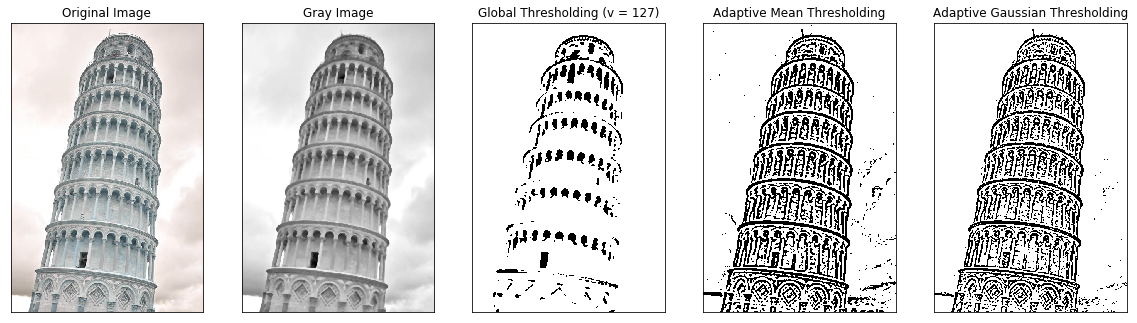## 四、Otsu二值化

• 在使用全局阈值时，我们就是随便给了一个数来做阈值，那我们怎么知道我们选取的这个数的好坏呢？答案就是不停的尝试。如果是一副双峰图像（简单来说双峰图像是指图像直方图中存在两个峰）呢？我们岂不是应该在两个峰之间的峰谷选一个值作为阈值？

• Otsu 二值化过程：对一副双峰图像自动根据其直方图计算出一个阈值。（对于非双峰图像，这种方法得到的结果可能会不理想）。

• 这里用到到的函数还是 cv2.threshold()，但是需要多传入一个参数（flag）：cv2.THRESH_OTSU。这时要把阈值设为 0。然后算法会找到最优阈值，这个最优阈值就是返回值 retVal。如果不使用 Otsu 二值化，返回的retVal 值与设定的阈值相等。

# 给图像添加噪声
def sp_noise(image,prob):

'''
添加椒盐噪声
prob:噪声比例
'''

output = np.zeros(image.shape,np.uint8)
thres = 1 - prob

for i in range(image.shape):
for j in range(image.shape):
rdn = random.random()
if rdn < prob:
output[i][j] = 0
elif rdn > thres:
output[i][j] = 255
else:
output[i][j] = image[i][j]

return output

import cv2
import random
import numpy as np
from matplotlib import pyplot as plt

# 添加椒盐噪声，噪声比例为 0.02
img = sp_noise(img, prob=0.02)

# global thresholding
ret1,th1 = cv2.threshold(img,127,255,cv2.THRESH_BINARY)

# Otsu's thresholding
ret2,th2 = cv2.threshold(img,0,255,cv2.THRESH_BINARY+cv2.THRESH_OTSU)

# 中值滤波
blur = cv2.medianBlur(img,5)
ret3,th3 = cv2.threshold(blur,0,255,cv2.THRESH_BINARY+cv2.THRESH_OTSU)

# plot all the images and their histograms
images = [img, 0, th1, img, 0, th2, blur, 0, th3]
titles = ['Original Noisy Image','Histogram','Global Thresholding (v=127)','Original Noisy Image','Histogram',"Otsu's Thresholding",
'Median filtered Image','Histogram',"Otsu's Thresholding"]

# 这里使用了 pyplot 中画直方图的方法，plt.hist, 要注意的是它的参数是一维数组
# 所以这里使用了（numpy）ravel 方法，将多维数组转换成一维，也可以使用 flatten 方法

plt.figure(figsize = (15, 10))
for i in range(3):
plt.subplot(3,3,i*3+1),plt.imshow(images[i*3],'gray')
plt.title(titles[i*3]), plt.xticks([]), plt.yticks([])
plt.subplot(3,3,i*3+2),plt.hist(images[i*3].ravel(),256)
plt.title(titles[i*3+1]), plt.xticks([]), plt.yticks([])
plt.subplot(3,3,i*3+3),plt.imshow(images[i*3+2],'gray')
plt.title(titles[i*3+2]), plt.xticks([]), plt.yticks([])

plt.show()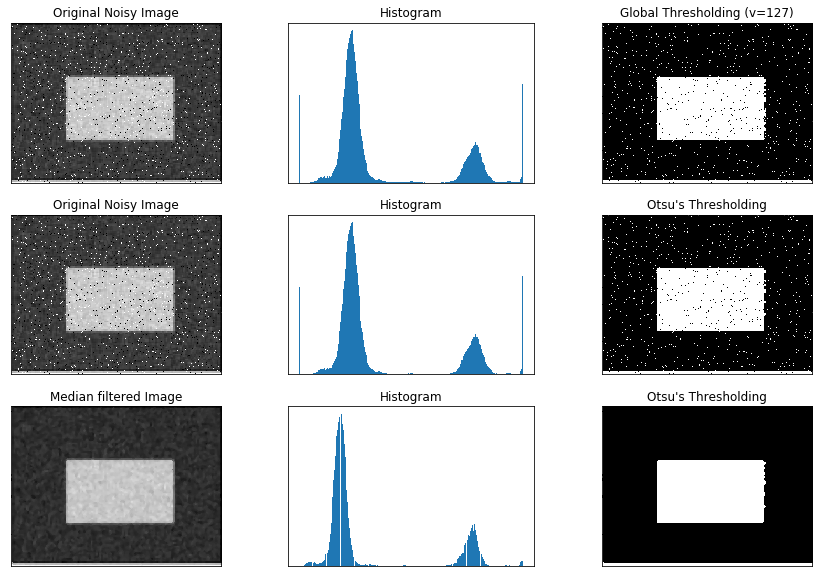## Otsu’s 二值化是如何工作的？

[外链图片转存失败,源站可能有防盗链机制,建议将图片保存下来直接上传(img-cIehSmuv-1590288463840)(attachment:image.png)]

import cv2
import numpy as np
from matplotlib import pyplot as plt

blur = cv2.GaussianBlur(img,(5,5),0)

# 计算归一化直方图
hist = cv2.calcHist([blur],,None,,[0,256])
hist_norm = hist.ravel()/hist.max()
Q = hist_norm.cumsum()

bins = np.arange(256)

fn_min = np.inf
thresh = -1

for i in range(1,256):
p1,p2 = np.hsplit(hist_norm,[i]) # probabilities
q1,q2 = Q[i],Q-Q[i] # cum sum of classes
b1,b2 = np.hsplit(bins,[i]) # weights

# finding means and variances
m1,m2 = np.sum(p1*b1)/q1, np.sum(p2*b2)/q2
v1,v2 = np.sum(((b1-m1)**2)*p1)/q1,np.sum(((b2-m2)**2)*p2)/q2

# calculates the minimization function
fn = v1*q1 + v2*q2
if fn < fn_min:
fn_min = fn
thresh = i

#print (thresh,ret)

ret, otsu = cv2.threshold(blur,130,255,cv2.THRESH_BINARY+cv2.THRESH_OTSU)

# 显示图像
titles = ['Input', 'blur','otsu']
images = [img, blur, otsu]

plt.figure(figsize = (20, 15))
for i in range(3):
plt.subplot(1,3,i+1)
plt.imshow(images[i],'gray')
plt.title(titles[i])
plt.xticks([]),plt.yticks([])
plt.show()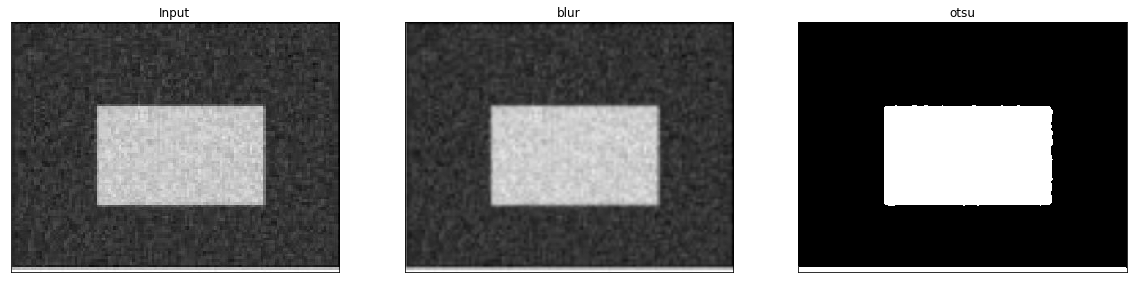## 参考文献

1. https://blog.csdn.net/qq_37385726/article/details/82015545
2. https://www.cnblogs.com/GaloisY/p/11037350.html
3. https://blog.csdn.net/sinat_36264666/article/details/77586964
4. https://blog.csdn.net/abcvincent/article/details/78822191
5. https://blog.csdn.net/hh555800/article/details/42342687
03-305494
12-05404
01-101891
01-061864
11-142924
07-031万+
01-2534
10-191万+
10-243615
05-082717
03-224257
11-151124
06-061903
08-131万+

### “相关推荐”对你有帮助么？

•非常没帮助
•没帮助
•一般
•有帮助
•非常有帮助被折叠的  条评论 为什么被折叠?到【灌水乐园】发言¥2 ¥4 ¥6 ¥10 ¥20余额支付 (余额：-- )扫码支付获取中扫码支付点击重新获取扫码支付1.余额是钱包充值的虚拟货币，按照1:1的比例进行支付金额的抵扣。
2.余额无法直接购买下载，可以购买VIP、C币套餐、付费专栏及课程。余额充值